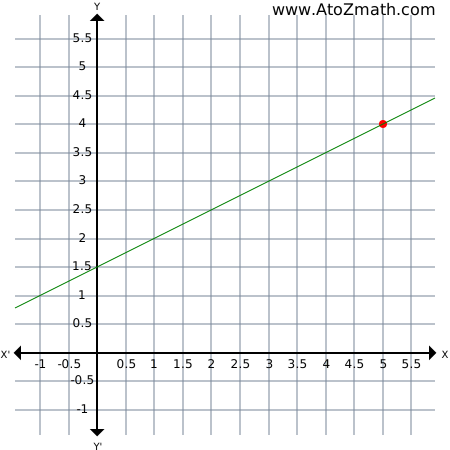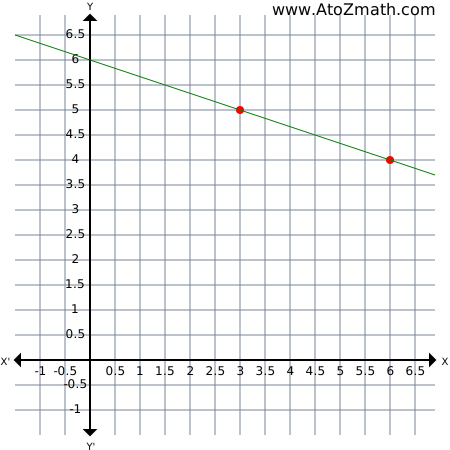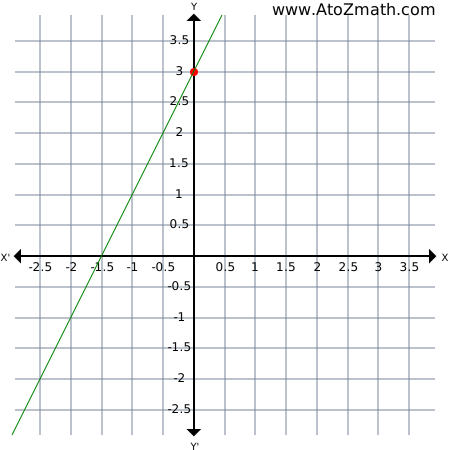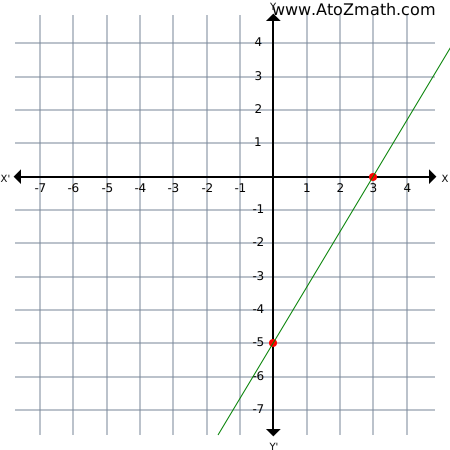Home > Geometry calculators > Coordinate Geometry > Find the equation of a line having slope = 2 and Y-intercept = 3 example

 6. Find the equation of a line using slope, point, X-intercept, Y-intercept example ( Enter your problem ) 1. Examples 1. Find the equation of a line having slope =1/2 and passing through point A(5,4)Solution:The equation of a line with slope m and passing through (x_1,y_1) is y-y_1=m(x-x_1)Putting (5,4) for (x_1,y_1) and m=1/2, we get:. y-4=1/2(x-5):. 2(y-4)=(x-5):. 2y -8=x -5:. x-2y+3=02. Find the equation of a line passing through two points A(3,5) and B(6,4)Solution:Here A(3,5), B(6,4) are the given points:. x_1=3, y_1=5, x_2=6, y_2=4The equation of a line AB is(y-y_1)/(y_2-y_1)=(x-x_1)/(x_2-x_1):. (y-5)/(4-5)=(x-3)/(6-3):. (y-5)/(-1)=(x-3)/(3):. 3(y-5)=-(x-3):. 3y -15=-x +3:. x+3y-18=03. Find the equation of a line having slope =2 and y intercept =3Solution:Equation of a line with slope m and intercept c on Y-axis is y=mx+cHere m=2 and c=3y=2x+3:. 2x-y+3=04. Find the equation of a line having X-intercept =3 and Y-intercept =-5Solution:Here intercept on X-axis a=3 and Y-intercept b=-5Equation of a line isx/a+y/b=1:. x/(3)+y/(-5)=1:. (x)/(3)-(y)/(5)=1:. 5x-3y=15This material is intended as a summary. Use your textbook for detail explanation.Any bug, improvement, feedback then Submit Here

Copyright © 2019. All rights reserved. Terms, Privacy

We use cookies to improve your experience on our site and to show you relevant advertising. By browsing this website, you agree to our use of cookies. Learn more